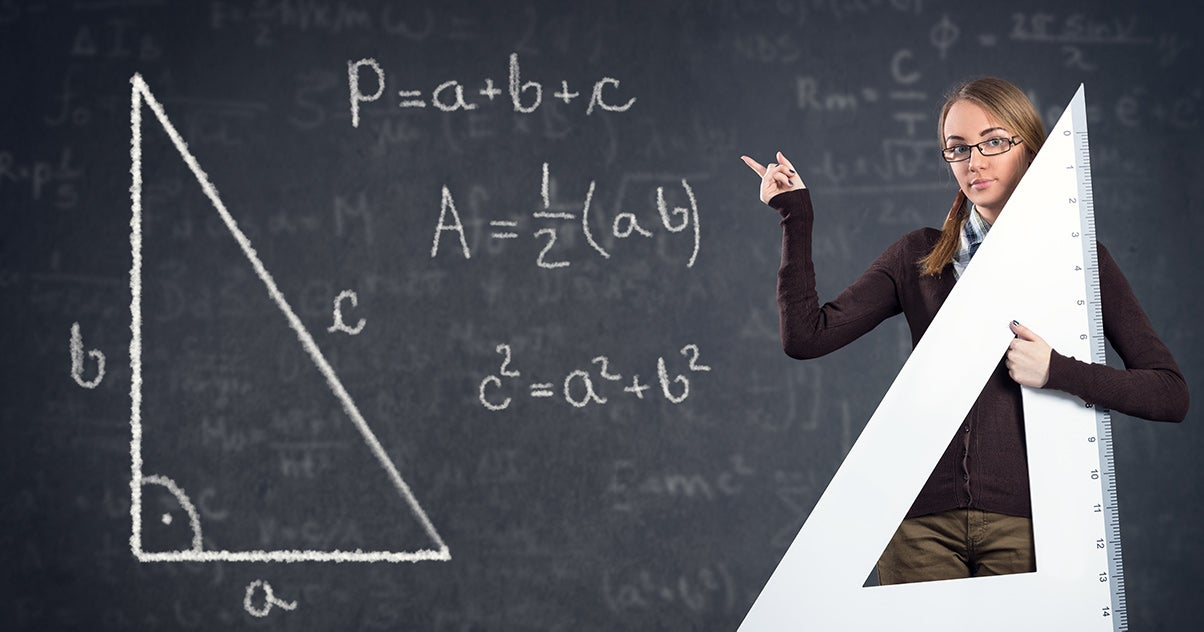Tips for Teachers and Classroom Resources

# Algebra: 3 High School Math Lesson Plans

By The Editorial Team

One of the biggest challenges high-school math teachers face is student apathy. However, by showing students the relationship between algebra and possible future careers or life situations, students will become more engaged and will better retain the information.

The following three lesson plans focus on teaching high school math skills in ways that relate to the student’s life.

## The Pythagorean Theorem

The Pythagorean Theorem is a hypothesis that explains the relationship between the three sides of a right angle triangle. To get started, you should remind students that the hypotenuse is the longest side of the triangle and that it is the side that is opposite from the 90 degree angle. The formula is a2 + b2 = c2. In this equation, a and b are the two short sides of the triangle while c is the hypotenuse. Alternatively, students may use the following formula: (side)2 + (side)2 = (hypotenuse)2.

Example one

1. Find a square or rectangle object for the students to evaluate. This object also could be an actual right angle triangle.
2. Have the students measure two sides of the object.
3. Direct the students to use their measurements to calculate the length of the side they have not measured.
4. Alternate which sides the students measure.

Example two

1. Have the students think of real-life problems when they may need this equation.
2. If the students cannot think of any examples, you should have them imagine a tent.
3. Ask the students to calculate whether or not they could sleep diagonally in a three-foot by four-foot tent. Next, have students calculate, based on their individual heights, the smallest size tent in which they could lie down.

## Velocity and force

In this lesson plan, students will explore the concepts of velocity and force. When isolated in formulas, these concepts can be difficult to understand, but when applied to real-life scenarios, they become more manageable for students.

Velocity is distance divided by time. When most students finish their algebra classroom homework, they will have memorized the equation v=d/t, but few of them will recall how to apply it.

Force can be computed in a number of ways, but for this algebra classroom homework, it will be computed by multiplying mass by distance times velocity divided by distance times time.

To initiate a discussion of velocity, teachers should reference a real-life experience. For instance, they may talk about a student on a skateboard running into a teacher, and they may discuss how much velocity the skateboarder would need to knock down the teacher.

To simulate the skateboarder, have students measure a softball rolling down a slope. Have students time the softball as it runs down the slope, then have them measure the distance of the slope. The distance divided by the time is the velocity of the ball. Ask the students to imagine whether or not velocity would increase as the length of the slope increases. Once students understand this, you can have them solve the equation for force using the following equation: F=m(dv/dt).

## Determining age

In this lesson, students will learn how to place verbal clues into simple equations to calculate a speaker’s age. The teacher should give a number of clues that may simulate what students may be likely to overhear in the real world concerning age, and then the teacher should have the students calculate the speaker’s age based on the clues provided.

For instance, the teacher could say: I was 26 when my son was born. My son was four when my daughter was born, and now, my daughter is four. How old am I?

To set up this formula, have students assign a letter to each character. For instance, t= teacher, s= son, and d= daughter. Then, have students arrange the letters into a number of equations with the information they have. They should come up with the following equations:

• t=s+26
• s=d+4
• d=4

With the information provided, students should be able to calculate these equations to find the age of the speaker.